# Subtraction up to 59

## NUMBERS AND OPERATIONSSubtraction is an arithmetic operation that is represented by a "minus" sign (-) and symbolizes the operation of removing objects from a collection.

It fulfills the property of the neutral element:

Any natural number subtracted by the number zero (0) results in the same number; for example: 8 - 0 = 8

It fulfills the distributive property:

The result of the subtraction of two numbers (A and B) multiplied by a third number (C) will be equivalent to the subtraction of their products (A x C - B x C); for example: 2 x (6 - 3) = 2 x 6 - 2 x 3

Subtraction is anti-commutative

A modification in the order of its elements (minuend and subtrahend) will modify the sign of the answer; for example: 6 - 4 ≠ 4 - 6.

Subtraction is non-associative

When three or more numbers are subtracted, the order in which the subtraction is performed always influences; for example: 5 - (3 - 2) ≠ (5 - 3) - 2.

What is subtraction for children and how do we teach it?

We are going to learn to solve subtractions with the natural numbers from 0 to 59 using as strategies the counting of units and the subtraction of the elements of a set. Also, enjoy the explanations that accompany the video that we propose.

SPANISHENGLISHTo understand correctly what a subtraction is, we provide you with educational worksheets where you have to count the number of elements in each set (either mentally or by hand) and check the result obtained when we remove or extract a certain amount:Therefore, we can say that "subtract" consists of removing, reducing or separating a part of the elements from an initial collection.

What do the symbols "-" and "=" mean in a subtraction?

From the 15th century, the current mathematical signs that represent the subtraction operation began to be used in Europe:

- The symbol "-" is read "minus", "less" or "subtracted from" and is always inserted between the elements that we have to subtract.

- The symbol "=" is read "equals", "equal", "is" or "are" and represents the result that we obtain after reducing, separating or extracting the elements of a total set into a new set.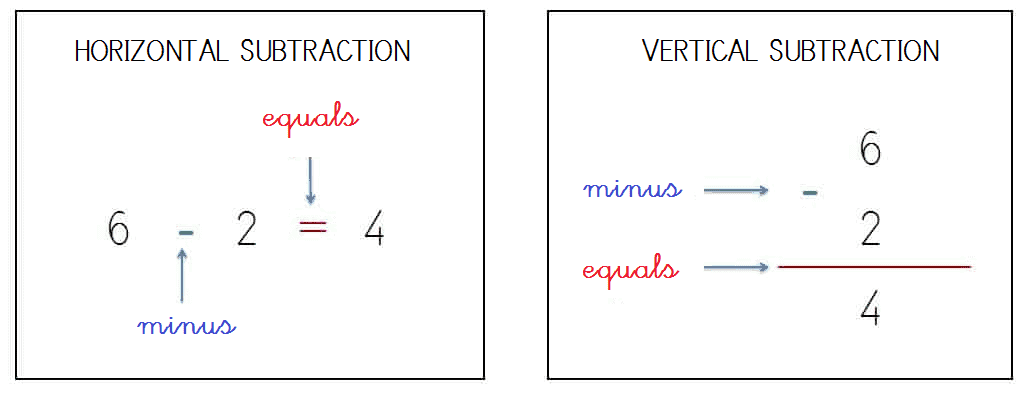#### Know the elements of subtraction and their position with natural numbers up to 59

What is the "minuend", the "subtrahend" and the "difference" in a subtraction?

Once we have recognized and interpreted the meaning of the mathematical symbols "-" e "=", we will explain in detail what the subtraction terms are:

- Minuend: Represents the total collection or initial set of elements.

- Subtrahend: Symbolizes the number or quantity of elements in which the minuend will be reduced.

- Difference or Rest: It corresponds to the unique set formed by separating or reducing the amount of the subtrahend on the minuend.

SPANISHENGLISH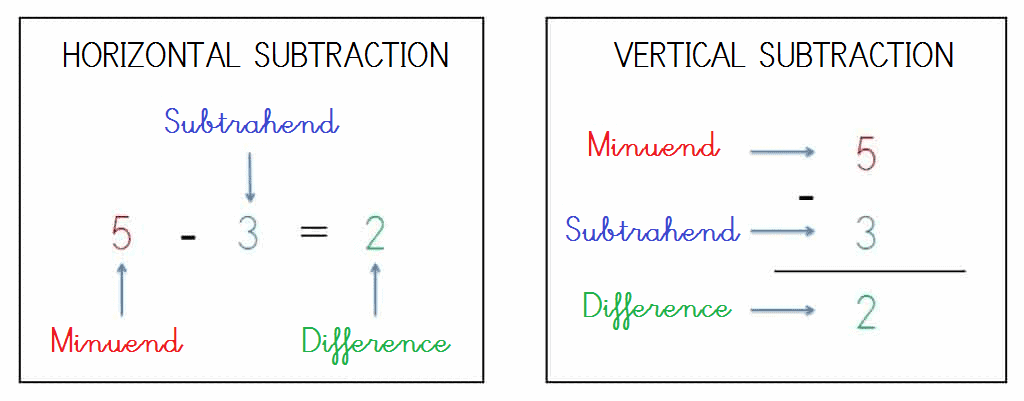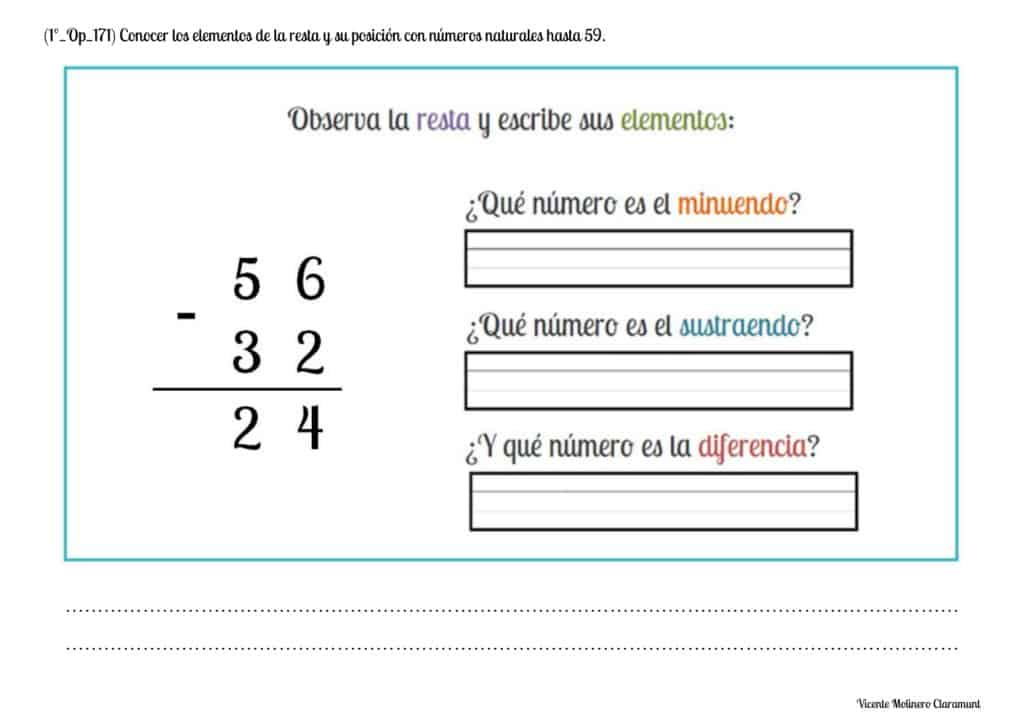#### Properly place the subtraction terms in natural numbers up to 59

Next you will learn to construct vertical subtractions placing the symbols "-" e "=" and each of its terms (minuend, subtrahend and difference) in the corresponding place practicing with the different printable educational worksheets that we have elaborated for the natural numbers from 0 to 50 following the method based on vertical columns.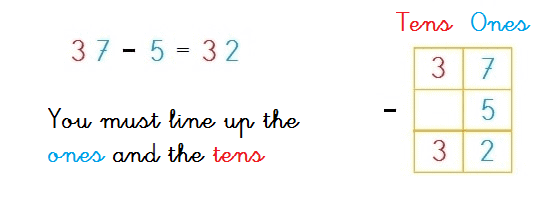#### Solve subtractions of two elements vertically without regrouping with whole numbers up to 59

How to subtract two-digit numbers without borrowing?

We present you some printable PDF activities accompanied by their explanation and an educational game so that you can easily learn how to subtract numbers from 0 to 60 vertically and without exchanging.

SPANISHENGLISH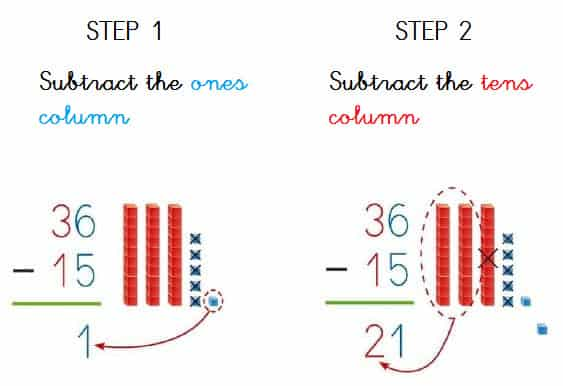#### Solve subtractions of two elements vertically with regrouping with whole numbers up to 59

We are going to explain how to solve any two-digit subtraction by regrouping using the the most taught methods in school: Austrian and American Method.

SUBTRACT BY COMPENSATION

SPANISHENGLISHThe Austrian Method (also called "by compensation") proposes the resolution of subtractions with borrowing "adding units to both the minuend and the subtrahend" when the minuend figure is less than the digit of the subtrahend: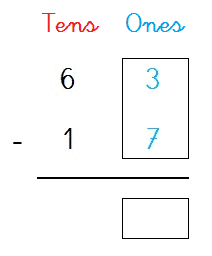1st) Can we subtract 3 - 7 in the ones column?

No, because 3 is less than 7 ( 3 < 7 ), therefore, we must ask for help in the tens column.

2nd) We add 1 ten to the subtrahend figure and 10 units to the minuend figure to compensate by equivalence the place value of its figures:

Subtrahend:

1 ten + 1 ten = 2 tens

Minuend:

3 ones + 10 ones (1 ten) = 13 ones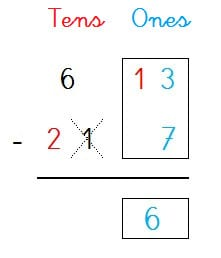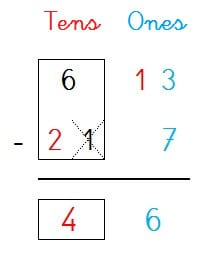3rd) We subtract the ones column because 13 is greater than 7:

13 - 7 = 6

4th) Finally, we will subtract the tens column:

6 - 2 = 4

5th) We already have the solution!

63 - 17 = 46

SUBTRACT BY GROUPING

SPANISHENGLISHThe American Method (also called "by grouping") proposes the resolution of the subtractions "by borrowing units between the minuend figures from left to right":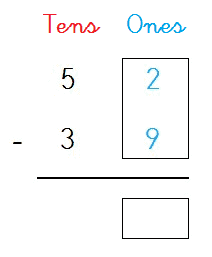1st) Can we subtract 2 - 9 in the ones column?

No, because 2 is less than 9 ( 2 < 9 ), therefore, we must ask for help in the tens column.

2nd) We reduce 1 ten of the minuend figure (tens column) and add it to the minuend figure of the ones column taking into account that 1 ten is equal to 10 ones:

Minuend (tens column):

5 tens - 1 ten = 4 tens

Minuend (units column):

2 ones + 10 ones (1 ten) = 12 ones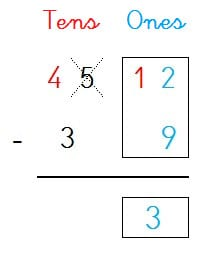3rd) We subtract the ones column because 12 is greater than 9:

12 - 9 = 3

4th) Finally, we will subtract the tens column:

4 - 3 = 1

5th) We already have the solution!

52 - 39 = 13#### Solve subtractions of two elements horizontally without regrouping with whole numbers up to 59

Learn to solve horizontal subtractions without borrowing with whole numbers from 0 to 60 with our didactic exercises starting from the largest number and then jumping bakward on a number line (either real or imaginary).#### Solve subtractions of two elements horizontally with regrouping with whole numbers up to 59

Now you have mastered the subtractions with borrowing vertically... Do you dare to solve them also horizontally? To help you, we give you a mathematical trick, "both vertical and horizontal subtractions are solved in the same way"... Think, push yourself and see how you get it!#### Solve subtractions of three elements vertically without regrouping with whole numbers up to 59

How to subtract 3 or more numbers without borrowing?

1) We will align the numbers according to the place value of their figures, that is, we will align the ones (1s) with the ones and the tens (10s) with the tens.

2) For the ones (1s), we will select a pair of numbers and subtract them from each other (for example, 9 - 3 = 6) and, from the result, we will subtract the third number (6 - 2 = 4).

3) We will repeat the same process for the tens (10s), we will select a pair of numbers and subtract them from each other (4 - 1 = 3) and, to the difference obtained, we will subtract the third digit (3 - 2 = 1).#### Mentally solve simple subtraction facts with natural numbers up to 59

Show your logical-mathematical skills by solving our exercises with hidden or missing whole numbers and using mental calculation (also known as "mental math").#### Solve subtraction word problems without regrouping with whole numbers up to 59

We have made educational cards so that you can practice solving subtraction problems without borrowing with the whole numbers from 0 to 60.

To make it easier for you, we provide you a step-by-step explanation through a video tutorial with 2 digit word problems without regrouping.

SPANISHENGLISH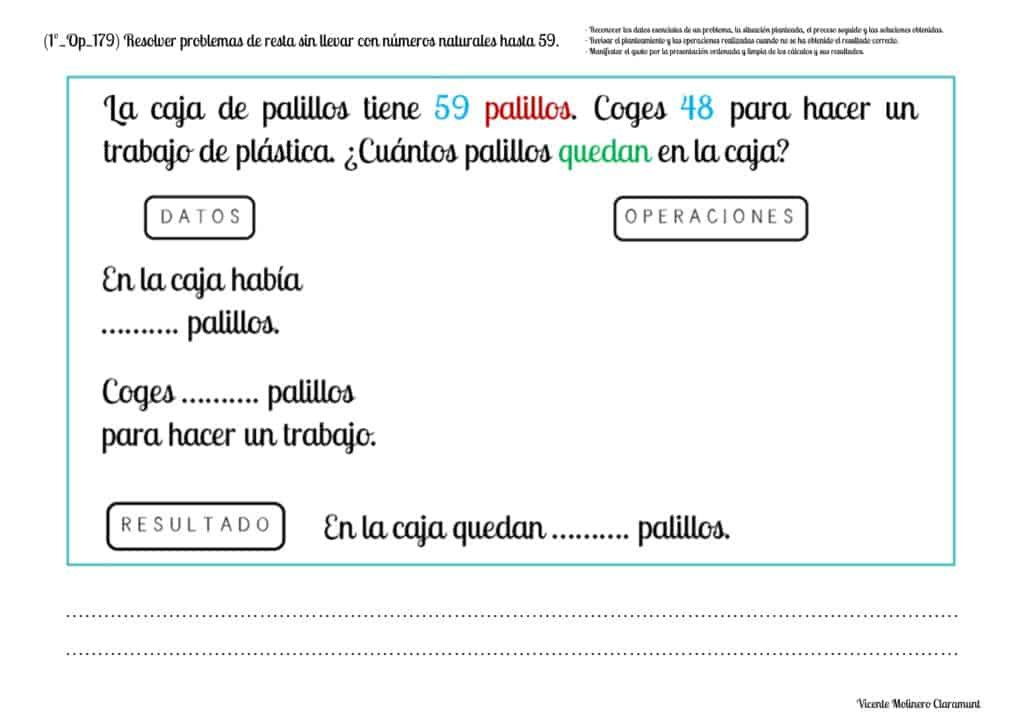#### Solve subtraction word problems with regrouping with whole numbers up to 59

Show that you are a math genius by solving each of our arithmetic problems using the basic operation of subtraction by borrowing from 0 to 60.

If you need a little preparation, watch our video lessons where we provide you with some solved examples using the column method.

SPANISHENGLISHSelect more exercises, worksheets and activities of Mathematics for each of the 4 learning blocks of the Educational Curriculum ("Numbers and Operations", "Quantities and Measurements", "Geometric and Spatial Reasoning" and "Data Analysis and Probability") aimed at improving the logical-mathematical competencies and skills that are developed throughout "Grade 1" or "Year 2":